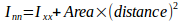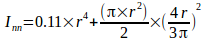## Q.1. Define Moment of Inertia and state its SI unit.

ANS : Moment of inertia is defined as,

Second moment of an area about an axis is called Moment of inertia.”

or

A quantity expressing the body’s tendency to resist angular acceleration, it is equal to sum of product of mass of particles to the square of distances from the axis of rotation.”

Moment of inertia =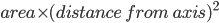SI unit of moment of inertia is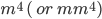## Q.2. Define radius of Gyration.

ANS : Moment of inertia is defined as,

Radius of gyration of a body about an axis is a distance such that when square of that distance is multiplied by the area of that body gives Moment of inertia of that body.”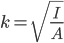## Q.3. State Parallel axis theorem.

It states that,

The moment of inertia of a lamina about any axis parallel to the centroidal axis is equal to the Moment of inertia of the body about its centroidal axis plus the product of the area and square of distance between these two axes.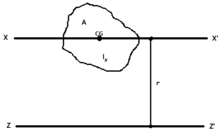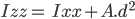## Q.4. State Perpendicular axis theorem.

It states that,

The moment of inertia of a lamina about an axis perpendicular to plane of lamina about and axis perpendicular to the lamina and passing through its centroidal is equal to sum of its moment of inertia about two mutually perpendicular axes lying in the plane.

## Q.5. Define Polar moment of Inertia.

It is defined as the moment of inertia of body about its centroidal axis which is perpendicular to the plane of the body.”

## Q.5. Write the equation for MI of Hollow circular section.

Ans: Moment of inertial of a hollow circular section is given by ,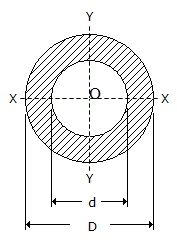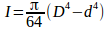D = Outer diameter of Hollow cylinder

d= Inner diameter of internal cylinder

## Q.6. Write the equation for MI of Hollow rectangular section.

Ans: Moment of inertial of a hollow circular section is given by ,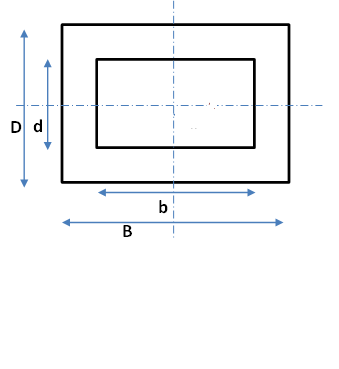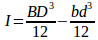B = Outer width of section

b= Inner width of section

D = Outer depth of section

d = Inner depth of section

## Q.7. Write the equation for MI of triangle about its base and apex.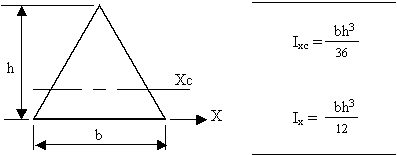## Q.8. Write the equation for MI of semicircle about its base.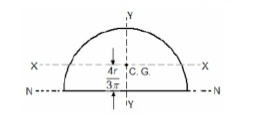Ans :

Moment of inertia about centroidal axis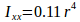Using parallel axis theorem